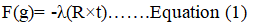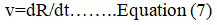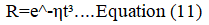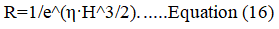All submissions of the EM system will be redirected to Online Manuscript Submission System. Authors are requested to submit articles directly to Online Manuscript Submission System of respective journal.

# Perspective

, Volume: 11( 6)

## A Mathematical Proof Of the "Big Crunch"

*Correspondence:
Department of Physics, Mahishadal Raj College West Bengal, India
Tel: 6295454694
E-mail: hh1564465@gmail.com

Received: June 14, 2023, Manuscript No. TSPA-23-102457; Editor assigned: June 16, 2023, PreQC No. TSPA-23-102457 (PQ); Reviewed: June 30, 2023, QC No. TSPA-23-102457; Revised: August 14, 2023, Manuscript No. TSPA-23-102457 (R); Published: August 21, 2023, DOI: 10.37532/2320-6756.2023.11(6).344

Citation: Sarkar NS. A Mathematical Proof of the “Big Crunch”. J Phys Astron. 2023;11(6):344.

### Abstract

In this paper I basically prove that the big crunch is not a hypothesis, but a reality. Although the big crunch hasn't happened yet. We have made many hypotheses about the death of the universe, but we have not found their correct theoretical proof. But my equation is able to mathematically describe the big crunch. This tells about a property known as the Hubble construction rate. As the universe increases in time, its size decreases exponentially. On the other hand, as the time increases again, the hubble construction rate will increase.

## Keywords

System; Universe; Big crunch; Hubble construction rate; Repulsive gravity

## Introduction

We are talking about the repulsive effect of gravity. As I have shown in my previous paper (Journal of physics and astronomy/volume 10, issue 10), gravity not only attracts everything, but acts as a kind of repulsive force on a large scale. And the equation is simple enough. A diagram I was trying to show in that paper, where a sphere was considered. A distribution of some amount of matter towards the center of the sphere was shown. And they had overall M mass. Then a single mass of matter at the extreme end of the sphere was considered. Then the gravitational force of the single mass (set, m=1) matter with M mass will be equal to –λ (R × t). Where R is the distance between matter of unit mass (m=1) and matter of mass M. t is the time acting on the system (in this case the system is the sphere). And λ is the repulsive gravitation constant.

## Description

Now think that the system that we have considered is actually expanding or contracting. So R will act as a kind of physical distance. Where R=a·Δx (where a is the scale factor and Δx is the coordinate distance which is always constant or fixed).In this case F(g)=GM/r² (general gravity) and since we have taken m=1, in the expression of F(g), there will be no small m. Now let's see a little Newtonian mechanics. According to Newtonian force:a' here acts as acceleration.

Now comparing the left side of equation (2) and equation (3) we will see that a' will be equal to GM/r². i.e.Substituting a' for GM/r² in equation (2), we get,Again we also know that,That is, acceleration is equal to the change in velocity with respect to time. Velocity on the other hand is equal to the change of distance with respect to time. i.e.By putting the value of v in equation (7), the value of v in equation (6), we get,Now substituting the value of a' in equation (8) into equation (5), we get:Or (d/dt)·(dR/dt)= -λ(R×t)

Or ∫d(dR/dt)= -λ·R·∫tdt

Or (dR/dt)= -λ·R·(t²/2)+c

Here c is a constant. Let c=0 for computational convenience i.e.

(dR/dt)= -λ·R·(t²/2)

Or ∫dR/R= -(λ/2) ∫t²dt

Or ln(R)= -(λ/6)t³+c'

In this case also c' is a constant. Let c'=0 for calculation convenience. And take λ/6=η i.e.

ln(R)= -ηt³

Or R=e^-(ηt³)Equation (10) says that as the time of the system or universe increases, its radius will decrease in the future. As the radius decreases it will eventually form a singularity. This mathematical description of mine shows the phenomenon called big crunch. That is, it will continue to decrease exponentially. Let's see some statistics of the mathematical expression we got according to equation (10).Derivative this equation with respect to t.

dR/dt= -3ηt²·e^-ηt³

Or Ṙ= -3ηt²·RSince R=e^-ηt³, Hence we can write equation (13) as:

Ṙ/R= -3ηt²

In this case v=Ṙ=ȧ·Δx means Again implying R=a·Δx.

Hence, v/R=Ṙ/R=ȧ/a=HIn this case H is the Hubble parameter. Now comparing equation (11) and (12) we get, H= -3ηt²

Or H²=9η²t⁴3η is a constant. I call it φ. So:Substituting the value of t in equation (15) into equation (10);Equation (16) says that the Hubble expansion rate is zero when time is zero. I would say that when the universe starts to contract, the Hubble expansion rate will work in reverse. That is then it will behave as Hubble contraction rate. In the same way that the Hubble expansion rate is related to the expansion of the universe, the Hubble contraction rate is related to the contraction of the universe. This contraction rate is capable of exponentially reducing the size of the universe which directly indicates big crunch. Time within the system is the same as it is now and will be in the distant future. The direction of time is always in the same direction so big crunch is not a time reversal process. There will come a time when the positive gravity of the matter present in the system will overcome the repulsive gravity and the size of the universe will decrease exponentially. This is the Hubble construction rate for which the big crunch will occur. Therefore, big crunch is not a hypothesis; rather it is a reality of nature.

## Conclusion

Finally, I can say that my contracting model is completely different from Friedman’s model. First I tried to show through repulsive gravity that the size of the universe is increasing, then by turning back the clock of the universe I was able to show that in the future the size of the universe will decrease rather than increase and at some point it will form the singularity. So the big crunch is indeed a reality. Here is the link of my previous paper “a theory on repulsive nature of gravity”.

Welcome Message

###### Citations : 291

Journal of Physics & Astronomy received 291 citations as per Google Scholar report

#### Indexed In

• Open J Gate
• China National Knowledge Infrastructure (CNKI)
• Cosmos IF
• Directory of Research Journal Indexing (DRJI)
• MIAR
• Secret Search Engine Labs
• Euro Pub
• clarivate-web-of-science-logo-vector
• ICMJE

View More